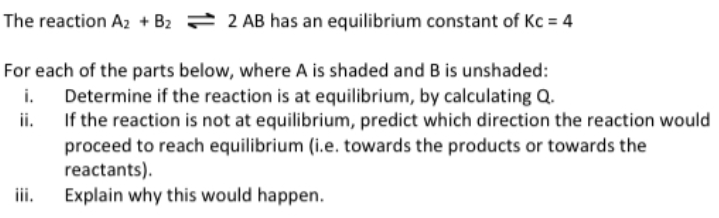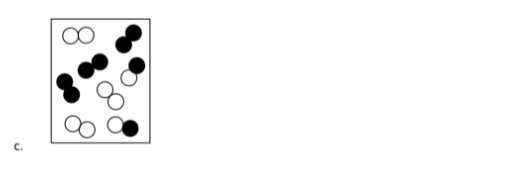# The reaction A2 + B2 ⇌ 2 AB has an equilibrium constant of Kc = 4 For each of the parts below, where A is shaded and B is unshaded: i. Determine if the reaction is at equilibrium, by calculating Q. ii. If the reaction is not at equilibrium, predict which direction the reaction would proceed to reach equilibrium (i.e. towards the products of towards the reactants). iii. Explain why this would happen.